# Isohedral figure

Isohedron redirects here. It is not to be confused with icosahedron.
For the related Isohedral numbers, see Anisohedral tiling.

In geometry, a polytope of dimension 3 (a polyhedron) or higher is isohedral or face-transitive when all its faces are the same. More specifically, all faces must be not merely congruent but must be transitive, i.e. must lie within the same symmetry orbit. In other words, for any faces A and B, there must be a symmetry of the entire solid by rotations and reflections that maps A onto B. For this reason, convex isohedral polyhedra are the shapes that will make fair dice.

Isohedral polyhedra are called isohedra. They can be described by their face configuration. A form that is isohedral and has regular vertices is also edge-transitive (isotoxal) and is said to be a quasiregular dual: some theorists regard these figures as truly quasiregular because they share the same symmetries, but this is not generally accepted.

A polyhedron which is isohedral has a dual polyhedron that is vertex-transitive (isogonal). The Catalan solids, the bipyramids and the trapezohedra are all isohedral. They are the duals of the isogonal Archimedean solids, prisms and antiprisms, respectively. The Platonic solids, which are either self-dual or dual with another Platonic solid, are vertex, edge, and face-transitive (isogonal, isotoxal, and isohedral). A polyhedron which is isohedral and isogonal is said to be noble.

## Examples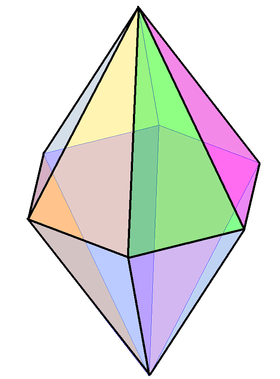The hexagonal bipyramid, V4.4.6 is a nonregular example of an isohedral polyhedron.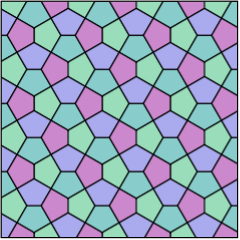The isohedral Cairo pentagonal tiling, V3.3.4.3.4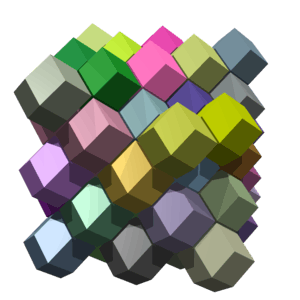The rhombic dodecahedral honeycomb is an example of an isohedral (and isochoric) space-filling honeycomb.

## k-isohedral figure

A polyhedron (or polytope in general) is k-isohedral if it contains k faces within its symmetry fundamental domain.

Similarly a k-isohedral tiling has k separate symmetry orbits (and may contain m different shaped faces for some m < k).

A monohedral polyhedron or monohedral tiling (m=1) has congruent faces, as either direct or reflectively, which occur in one or more symmetry positions. An r-hedral polyhedra or tiling has r types of faces (also called dihedral, trihedral for 2 or 3 respectively).

Here are some example k-isohedral polyhedra and tilings, with their faces colored by their k symmetry positions:

3-isohedral 4-isohedral isohedral 2-isohedral
(2-hedral) regular-faced polyhedra Monohedral polyhedra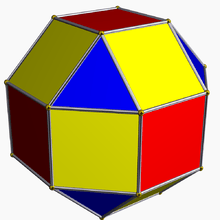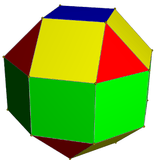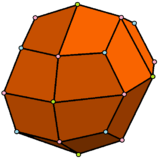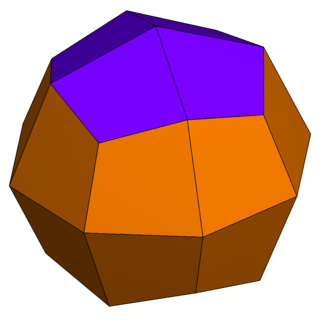The rhombicuboctahedron has 1 type of triangle and 2 types of squares The pseudo-rhombicuboctahedron has 1 type of triangle and 3 types of squares. The deltoidal icositetrahedron has with 1 type of face. The pseudo-deltoidal icositetrahedron has 3 types of identical-shaped faces.
2-isohedral 4-isohedral Isohedral 3-isohedral
(2-hedral) regular-faced tilings Monohedral tilings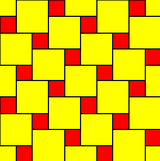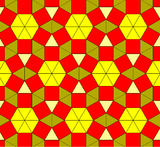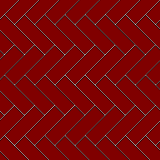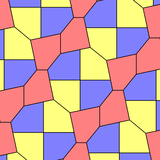The Pythagorean tiling has 2 sizes of squares. This 3-uniform tiling has 3 types identical-shaped triangles and 1 type of square. The herringbone pattern has 1 type of rectangular face. This pentagonal tiling has 3 types of identical-shaped irregular pentagon faces.

## Related terms

A cell-transitive or isochoric figure is an n-polytope (n>3) or honeycomb that has its cells are congruent and transitive with each other.

A facet-transitive or isotopic figure is a n-dimensional polytopes or honeycomb, with its facets ((n-1)-faces) congruent and transitive. The dual of an isotope is an isogonal polytope. By definition, this isotopic property is common to the duals of the uniform polytopes.

• An isotopic 2-dimensional figure is isotoxal (edge-transitive).
• An isotopic 3-dimensional figure is isohedral (face-transitive).
• An isotopic 4-dimensional figure is isochoric (cell-transitive).

## Notes

1. McLean, K. Robin (1990), "Dungeons, dragons, and dice", The Mathematical Gazette, 74 (469): 243–256, JSTOR 3619822.
2. Socolar, Joshua E. S. (2007). "Hexagonal Parquet Tilings: k-Isohedral Monotiles with Arbitrarily Large k" (corrected PDF). The Mathematical Intelligencer. 29: 33–38. doi:10.1007/bf02986203. Retrieved 2007-09-09.
3. Craig S. Kaplan. "Introductory Tiling Theory for Computer Graphics". 2009. Chapter 5 "Isohedral Tilings". p. 35.
4. Tilings and Patterns, p.20, 23

## References

• Peter R. Cromwell, Polyhedra, Cambridge University Press 1997, ISBN 0-521-55432-2, p. 367 Transitivity